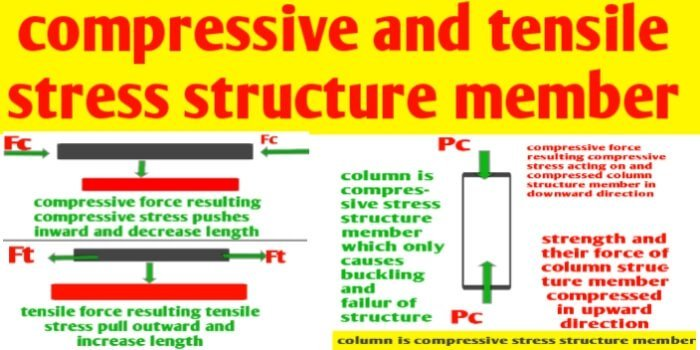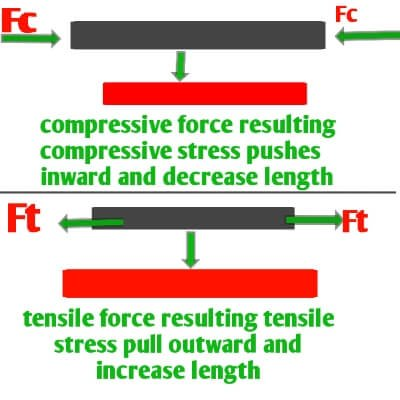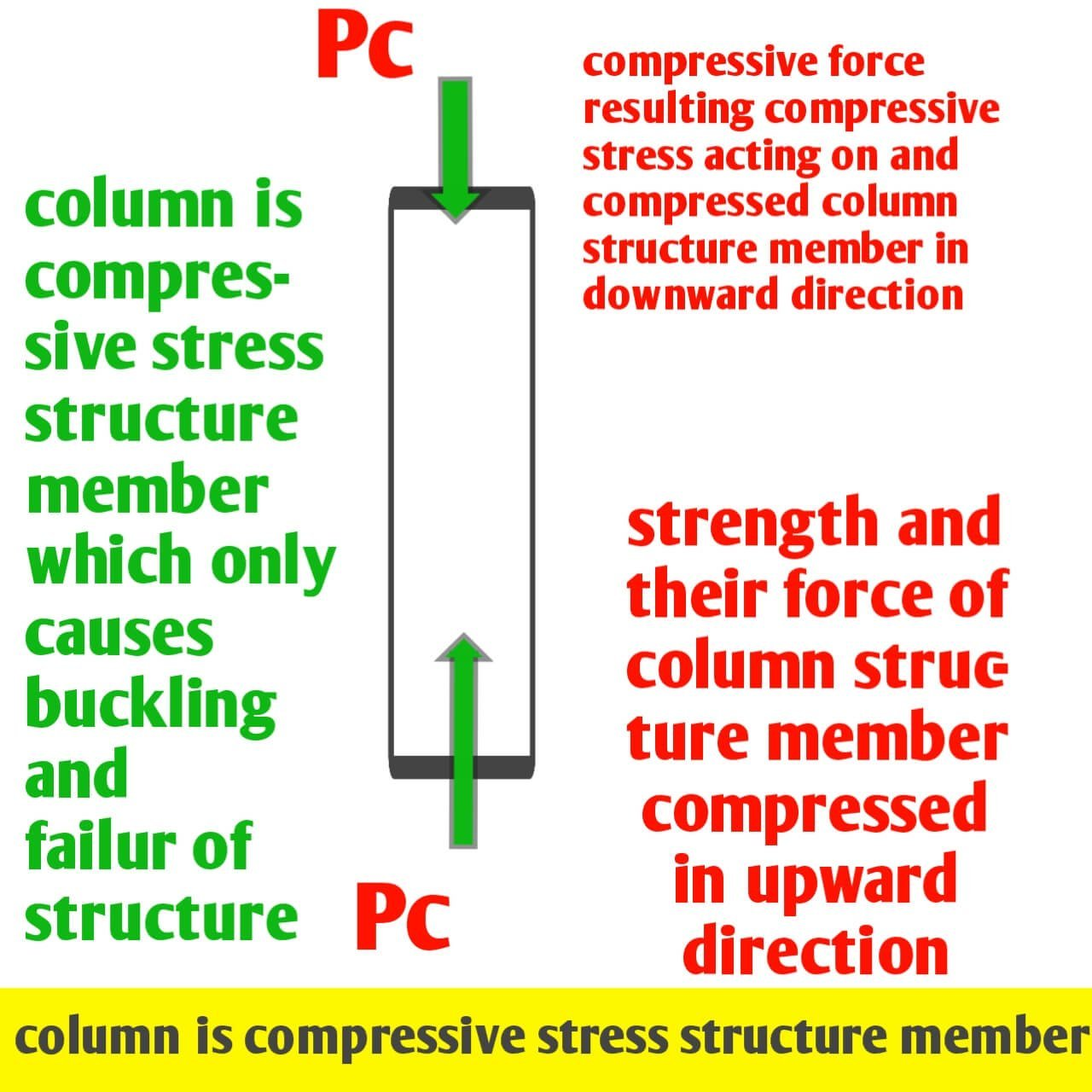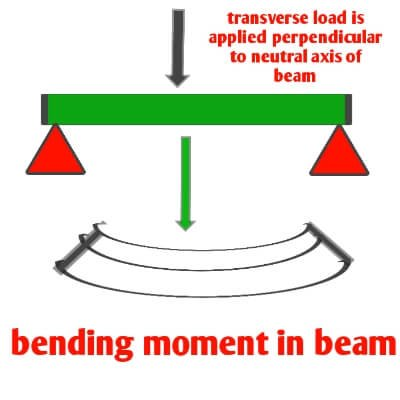# Compressive and tensile stresses in structural members

Compressive and tensile stresses in structural members

Introduction :- hi guys in this article we know about compressive and tensile stresses in structural members we know that in building designing we have several important structural member is like design of column, design of beam, design of slab and if trusses is provided for sloping slab. So we have many structural member like column, beam ,slab and trusses.

These are structural member influenced by various type of forces acting on it that causes development of stress like compressive stress and tensile stress. Compressive force acting on structural member resulting compressive stress which squeeze a member or compressed it and tension force acting on structural member is development of tensile stress which pull the structure members in tension. Now let us discuss about development of compressive stress and tensile stress in different structural members.

You should also visits:-

1)what is concrete and its types and properties

2) concrete quantity calculation for staircase and its formulaCompressive and tensile stresses in structural members

## What is stress and their typesCompressive and tensile stresses in structural members

Stress represents the action of force or moment on different types of structural members resulting and developing a stress which pull or pushes the structure member in outward direction or inward direction respectively. Stress which is developed in structural member is denoted by ( f ) and it is ratio of applied force (P) and area (A)of cross section of a structure member.

Stess = Acting force/Area

f = P/A and measured in N/m2

There is two types of stress one is negative stress that is compressive stress and second one is positive stress that is tensile stress.

1) Compressive stress : this type of stress is represented by fc. If the compressive forces acting on a structure member resulting compressive stress which pushes or compressed the structure by both end in inward direction that resulting decrease in length is known as compressive stress and compressive stress is squeeze a member.

Compressive stress (fc) is measured by the ratio of applied compressive force ( Pc) and area (A) of cross section of a structure member.

Compressive stress = compressive force/Area

fc = Pc/A and it is measured in N/m2

### Why compressive stress is considered as negative

The compressive force acting on structure members resulting compressive stress which compressed the structure and decreasing their length that’s why compressive force is taken as negative and vice versa also compressive stress is is negative.

There is decrease in length (strain) of a structure member due to compressive stress is taken as negative. Thus compressive stress results in the decrease of the length of member if the original length is l and the change in length is ∆l taken negative then

Compessive stress fc = (_ P)/A ——-(eq1)

Strain = change in length/original length

Strain = ( _ ∆l)/l ———-( eq2)

Properties of compressive stress

By developing compressive stress structure member have buckled or crushed and it will be either brittle and deformed. Buckling phenomenon is only occurs when a structure member is applied to compressive stress only and structure member have not properties of ductility in the case of compressive stress. And buckling does not occurs when a structure member is subjected to tensile stress.

2) Tensile stress : this type of stress is represented by ft. If the tensile forces acting on a structure member resulting tensile stress which pull or stretched the structure by both end in outward direction that resulting increased in length is known as tensile stress and tensile stress (ft) is measured by the ratio of applied tensile force ( Pt) and area (A) of cross section of a structure member.

Tensile stress = tensile force/Area

ft = Pt/A and it is measured in N/m2

### Why tensile stress is considered as positive

The tensile force acting on structure members resulting tensile stress which pull the structure in outerward direction and elongates in their length that’s why tensile force is taken as positive and vice versa also tensile stress is positive. There is increase in length (strain) of a structure member due to tensile stress is taken as positive.

Thus tensile stress results in the increasing of the length of member .if the original length is l and the change in length is ∆l taken positive then

Tensile stress ft = (+ P)/A ——-(eq1)

Strain = change in length/original length

Strain = ( + ∆l)/l ———-( eq2)

Properties of tensile stress

Tensile stress along with compressive stress causes bending moment. That type of force tension force and compressive force is applied on a structure member that’s resulting bending stress which is combination of both tensile stress and compressive stress. Thus bending stress is resulting bending of a structure member having ductility properties and this type of structure member do not brittle.

### Types of a structure members in building

According to development of compressive stress, tensile stress and bending stress in different structure members in building like column, beam, slab and trusses it is categorised into three types

1) compressive structural number

2) Flexural structural member

3) Axil structural member

### Why column is compressive structure numberCompressive and tensile stresses in structural members

We know that all the summary of load of building finally transfer to the the column to the bed of soil. All the horizontal loads of slab uniformly distributed and transfer to the beam and all the horizontal load acting on Beem it transfer vertically to the column.thus vertical load acting on column in downward direction try to resulting compressed in downward direction and vis versa supporting strength of column and their internal force counter with vertical load and acting in upward direction which also try to compress structure member column in upward direction.

So column structure member is compressed by both end like that one end is compressed in downward direction by vertical load acting on it and second end compressed by strength of column and their internal force in upward direction. Thus why column is acting as only compressive structure member having buckling properties.

#### What is flexural structure memberSagging positive bending momentHogging bending moment

#### Why beam and slab is flexural structure member

Different types of slab and beams is put together in the category of flexural structural member. When dead and live load acting on slab which transfer to beam resulting bending moment and bending of slab and beam which shows concavity in downward and convexity in upwarddirection.

Concavity in downward is known as sagging that is positive bending moment which has compression zone in top of slab and beam which is made of only concrete in slab and lesse quantity of reinforcement in beam. and tension zone in bottom of slab and beam which is made of reinforcement of conrete. And there is neutral axis between centroid of compression zone and tension zone of where bending moment is zero. Bending moment is results of both type of moment compressive stress and tensile stress that’s why slab and beam structure is put in category of flexural structure number.

#### What is axial structure member

Why trusses is axial structure memberStrut is compressive member of trusses

Trusses are typicy Framework consist of Rafter, post and struts which supporting roof in building ,bridges and other structure. When axial load is acting on joint of trusses than trusses is act as inherent geometric stability of the triangle to evenly distribute weight and to handle changing tension and compresson and the trusses uses a web of triangle that is strut are joined so that pressure and tension applied to the point of corner of each triangle take advantage of their stability to support of a structure by avoiding any bending and buckling of structure.

By connecting a series of trusses together and enormous amount of weight can be safely transfer to load bearing beam and wall without causing bending and buckling that’s why Trusses are put in category of axial structure member of building.